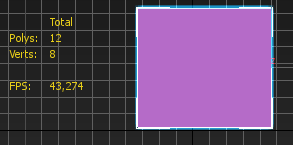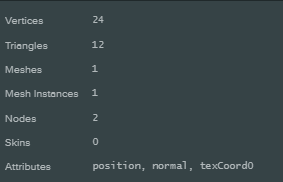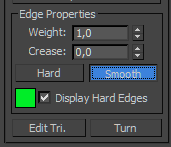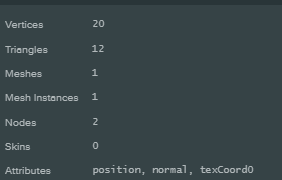# Wrong vertex count

Hello.

I have mesh in 3Ds max with 211 vertexes and 192 polys, but when I upload it to Editor, it increases to 410\880.

Why? I export my mesh to FBX. Maybe I have to check some options in exporter?

Thanks.

When you have an edge that is marked as sharp, 3Ds will count it as a single edge (two vertices) but every game engine will need two edges (four edges) to represent the corner.
This is because game engines work with vertices that have only a single normal, whereas most 3D modelling programs support split-normals.
If you have a manifold (4 faces per vert) mesh with every edge marked as sharp, then the polycount will quadruple when you convert it for a game engine. You clearly have some smooth and flat edges, so going from 211 -> 410 is reasonable.

1 Like

Okay, so I tested it with regular box without anythingI still don’t understand. Count of triangles is right - 6 poly * 2.

But why I have 24 vertices?

Also, If I set edges of my box to “smooth” I have 20 verticesHow? It doesn’t make sense for me…

It’s because each face needs a vertex at each corner to provide a normal for that face. So as each vertex is associated with 3 faces, there in fact need to be 3 vertices so that each one can have the correct normal.

Imagine the top left corner of a box. It has one location in real space, but there are three faces each with orthogonal normals that converge there. As a game engine requires one vertex per normal, you now need three points in the world at that location to provide a normal for the three faces which meet at that point. If you only had one vertex then it would have to have a normal that was the average of all three faces, clearly that would mess up lighting etc etc as it wouldn’t be pointing the right way for any face.

So a box requires “Sharp” edges. Something with smooth edges wants the normal to be the average. As @sdfgeoff says your model must have a mixture of sharp and smooth edges.

2 Likes

Okay, I thought engine interpolate between normal vector of each vertex of the face to calculate it’s normal.

So, when I display normals in 3ds max I see this

So each vector is a different point for engine, right?

And If I make part of model edges is smooth, it looks like some vectors disappear because of smooth interpolation between faces?

So my next question in this chain is when somebody says that my scene should be about 10’000 vertices which vertices does he mean?

Yes you’ve got it there. There are 24 blue normal lines in 3DS for the vertex normals (they are `split normals`), but that’s 24 vertices in the engine.

A 10k limit? That would be the ones actually used by the graphics card, so the larger number.

Thanks for explanation!

Yes, 10k limit is for old mobile devices.
I have to control my count in 3ds or engine?..

Well you are going to need to do it for the engine I guess, you really won’t know exactly the count until you import the models.

And ouch that’s a small limit isnt it :(((

Figuring my game with around 100k triangles isn’t going to play well on old mobiles!

Wow, 100k is a large number!

Did you test your game in mobiles? Which result?

It’s ok on modern stuff, (most of that is offscreen, I’m minimizing drawcalls which increases triangles due to less culling). Actually drawn on screen is a lot less - but they are being calculated.

So count large number of triangles influences only on memory amount allocated to scene?
If player see only small part of scene other triangles would be culled in render loop, right?

Well culling is pretty expensive at times, it’s a toss up. My level is made out of lots of small items with few verts placed around the scene. Walls/items on the floor etc. I’ve got a mesh combiner I wrote that bundles together everything with less than 300 verts into one big model. This is a good balance between CPU and GPU load I believe (based on what Unity does).

Mind you I also trade memory for performance on the synchronisation of the entity hierarchy by overriding the default way of working out all of the transformations and caching things in closures. Still hierarchy update time and cull time are seriously effected by lots of items.

The answer to this is really - which am I going to be bound by CPU/GPU on device X. Then make a choice of what to combine, how many triangles etc.

I’ve seen significant performance boosts on iPhone 5 by combining all of my animated characters into one huge mesh and then using bones to transform them outside of the camera clip (on the GPU) when not visible. So in other words technically rendering 20 things when perhaps only 5 are visible - still it was a lot faster than trying to get the CPU to do the clever stuff on culling.

Hm, nice trick! Did you test your app with and without it? Do you feel difference?

Oh yeah. It makes a huge difference. Massively reduces CPU load. I have 2 combiners. A static mesh combiner and a skinned mesh combiner. The skinned one does leave the hierarchy in place, so it’s only about draw call reduction. The static combiner drops the hierarchy for everything.

Happy to share it, but given my “webpack” etc ES6 stuff it will need work to get the dependencies and stuff for an ES5 project. It should provide the basic principles though.

It also does a “grid combine” where it divides a set of entities up into a grid and combines each cell of that grid, this way you still get some benefit of frustum culling.

``````import 'of-type'
import groupBy from 'lodash/groupBy'
import flatten from 'lodash/flatten'
import uniq from 'lodash/uniq'
import forEach from 'lodash/forEachRight'
import Promise from 'bluebird'
import asset from 'awaitassets'
import Defer from 'defer'
import debounce from 'lodash/debounce'

import {Q, V} from 'working'

const app = pc.Application.getApplication()

const VERTEX_LIMIT = 63000
const validTypes = {
"POSITION": true,
"NORMAL": true,
"TANGENT": true,
"TEXCOORD0": true,
"TEXCOORD1": true
}

function returnTrue() {
return true
}

function deleteObject(obj) {
if (obj.parent)
obj.parent.removeChild(obj)
obj.destroy()
}

let combinePromise = Promise.resolve(true)

function combining() {
let promise = combinePromise
let call = debounce(function call() {
if (promise === combinePromise) {
app.lightmapper.bake(null, app.scene.lightmapMode)
}
}, 2)
combinePromise.then(call)
}

predicate = predicate || returnTrue
let defer = new Defer()
combinePromise = combinePromise.then(() => defer.promise)
combining()
try {
cb = cb || deleteObject
const meshes = []

entity.ofType('model')
.filter(model => model.model && model.enabled && model.entity._enabled)
.forEach(model => {
model.meshInstances
.filter(instance => instance.visible !== false)
.forEach(instance => {
meshes.push({
mesh: instance,
material: instance.material,
model: model
})
})
})

await Promise.all(meshes.map(mesh => asset(mesh.model.asset)))

let byMaterial = groupBy(meshes, mesh => mesh.material.id)

let replace = new pc.Entity
replace.name = "Replace"
replace.enabled = true

let transform = new pc.Mat4()
let worldToLocal = new pc.Mat4()
worldToLocal.copy(entity.getWorldTransform())
worldToLocal.invert()
let models = []

forEach(byMaterial, list => {
if (!list.length) return
let material = list.material

let pos = []
let uv = []
let uv1 = []
let normal = []
let indices = []
let tangents = []
let fixups = []
let p = 0

function createCombinedMesh() {
let combined = new pc.Entity
combined.name = material.name + " Holder"
combined.enabled = true
combined.model.lightmapped = lightmapped
combined.model.lightmapSizeMultiplier = 128
combined.model.data.isStatic = true

//Fix up the UV1s
let side = Math.ceil(Math.sqrt(fixups.length))
let hside = Math.ceil(fixups.length / side)
let w = 1.0 / side
let h = 1.0 / hside
let x = 0
let y = 0
for (let i = 0; i < fixups.length; i++) {
fixups[i](x, y, w * 0.97, h * 0.97)
x += w
if (x >= 0.99) {
x = 0
y += h
}
}

fixups = []

let mesh = pc.scene.procedural.createMesh(app.graphicsDevice, pos, {
normals: normal,
uvs: uv,
uvs1: uv1,
indices: indices,
tangents: pc.calculateTangents(pos, normal, uv, indices)
})

let root = new pc.scene.GraphNode
let instance = new pc.scene.MeshInstance(root, mesh, material)
instance._aabb = mesh.aabb
let model = new pc.scene.Model
model.graph = root
model.meshInstances = [instance]
let asset = new pc.Asset('Combined Mesh ' + material.name, 'model')
asset.resource = model
combined.model.data.asset = asset.id
combined.model.data.type = 'asset'
combined.model.model = model
pos = []
uv = []
normal = []
indices = []
tangents = []
p = 0

return mesh
}

//Now loop through and transform everything
forEach(list, m => {
if (!predicate(m)) {
m.model.lightmapped = lightmapped
m.model.data.isStatic = true

return
}
//First get the world transform of the item
transform.copy(m.mesh.node.getLocalTransform());
let scan = m.mesh.node.getParent();
while (scan) {
transform.mul2(scan.getLocalTransform(), transform);
scan = scan.getParent();
}

// transform.copy(m.mesh.node.getWorldTransform())
// transform.mul(worldToLocal)

if (pos.length / 3 > VERTEX_LIMIT) {
createCombinedMesh()
}

let vb = m.mesh.mesh.vertexBuffer
let ib = m.mesh.mesh.indexBuffer[pc.RENDERSTYLE_SOLID]
let iblocked = ib.lock()
let indexes = new Uint16Array(iblocked)
let locked = vb.lock()
let format = vb.getFormat()
let base = m.mesh.mesh.primitive.base
let stride = format.size / 4
let data = {}
for (let j = 0; j < format.elements.length; j++) {
let element = format.elements[j]
if (validTypes[element.name]) {
data[element.name] = new Float32Array(locked, element.offset)
}
}
let positions = data.POSITION
let vec = new pc.Vec3()
let t = p

//Make room for the new ones
let verticesCount = Math.floor(positions.length / stride)
for (let i = 0; i < verticesCount; i++) {
pos.push(0)
pos.push(0)
pos.push(0)
uv.push(0)
uv.push(0)
uv1.push(0)
uv1.push(0)
tangents.push(0)
tangents.push(0)
tangents.push(0)
normal.push(0)
normal.push(0)
normal.push(0)
}
let tv
for (let i = 0; i < positions.length; i += stride) {
vec.set(positions[i], positions[i + 1], positions[i + 2])
tv = transform.transformPoint(vec, vec)
pos[t] = tv.x
pos[t + 1] = tv.y
pos[t + 2] = tv.z
t += 3
}
let normals = data.NORMAL
t = p
if (normals) {
for (let i = 0; i < normals.length; i += stride) {
vec.set(normals[i], normals[i + 1], normals[i + 2])
vec = transform.transformVector(vec, vec)
normal[t] = vec.x
normal[t + 1] = vec.y
normal[t + 2] = vec.z
t += 3
}

}
let uvs = data.TEXCOORD0
t = p / 3 * 2
if (uvs) {
for (let i = 0; i < uvs.length; i += stride, t += 2) {
uv[t] = uvs[i]
uv[t + 1] = uvs[i + 1]
}
}
let uvs1 = data.TEXCOORD1
t = p / 3 * 2
if (uvs1) {
for (let i = 0; i < uvs1.length; i += stride, t += 2) {
uv1[t] = uvs1[i]
uv1[t + 1] = uvs1[i + 1]
}
}
(function (p) {
fixups.push(function (x, y, w, h) {
let t = p / 3 * 2
//Fixup the uv1s
for (let i = 0; i < uvs1.length; i += stride, t += 2) {
uv1[t] = x + uv1[t] * w
uv1[t + 1] = y + uv1[t + 1] * h
}
})
})(p)
let numIndices = m.mesh.mesh.primitive.count

for (let i = 0; i < numIndices; i++) {
indices.push(indexes[i + base] + p / 3)
}
p += (positions.length / stride) * 3
//Turn off the existing object
models.push(m.model.entity)
vb.unlock()
ib.unlock()

})
createCombinedMesh()

})
replace.enabled = true
entity.simplify()
replace.setPosition(pc.Vec3.ZERO)
replace.setRotation(pc.Quat.IDENTITY)
models.forEach(cb)
return {replace, models}
} catch (e) {

} finally {
setTimeout(() => defer.resolve())
}
}

}

cols = cols || 4
rows = rows || 4
//First work out the world dimensions
let models = entity.ofType('model')
await Promise.all(models.map(model => asset(model.asset)))
let meshes = flatten(models
.filter(model => model.model && model.entity._enabled)
.map(model => model.meshInstances.map(mesh => ({mesh, model}))))
let aabb = new pc.BoundingBox
meshes.forEach(mesh => {
})
let holders = []
let min = aabb.getMin()
let max = aabb.getMax()
let width = Math.abs(min.x) + Math.abs(max.x)
let depth = Math.abs(min.z) + Math.abs(max.z)
let stepWidth = width / cols
let stepDepth = depth / rows
let dWidth = V(stepWidth, 0, 0).clone()
let dDepth = V(0, 0, stepDepth).clone()
let extents = V(stepWidth / 2, 1000, stepDepth / 2).clone()
let startCorner = V(min).Y(aabb.center.y).add(new pc.Vec3(dWidth / 2, 0, dDepth / 2)).clone()
for (let r = 0; r < rows + 1; r++) {
for (let c = 0; c < cols + 1; c++) {
let test = new pc.BoundingBox(centre, extents)
let usedMeshes = meshes.filter(mesh => !mesh.model._moved && mesh.mesh.aabb.intersects(test))
if (!usedMeshes.length) continue
let moveModels = uniq(usedMeshes.map(mesh => mesh.model))
let holder = new pc.Entity
holder.name = "Grid " + c + " x " + r
forEach(moveModels, model => {
let pos = V(model.entity.getPosition())
let rot = Q(model.entity.getRotation())
model.entity.parent.removeChild(model.entity)
model.entity.ofType('model').forEach(m => m._moved = true)
model.entity.setPosition(pos)
model.entity.setRotation(rot)
})
holder.syncHierarchy()
holders.push({
holder,
combined
})
}
}
console.log("Did not combine", meshes.filter(m => !m.model._moved).length)
meshes.filter(m => !m.model._moved).forEach(m => m.model.entity.destroy())
entity.simplify()
return holders
}